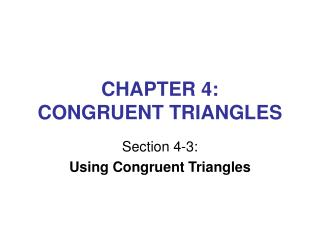DownloadDownload PresentationCHAPTER 4: CONGRUENT TRIANGLES

# CHAPTER 4: CONGRUENT TRIANGLES

Download Presentation## CHAPTER 4: CONGRUENT TRIANGLES

- - - - - - - - - - - - - - - - - - - - - - - - - - - E N D - - - - - - - - - - - - - - - - - - - - - - - - - - -
##### Presentation Transcript

1. CHAPTER 4: CONGRUENT TRIANGLES Section 4-3: Using Congruent Triangles

2. OBJECTIVE In the previous section, we studied different techniques for proving triangles congruent. In this section, we will deduce information about parts of triangles after we have shown the triangles to be congruent.

3. A WAY to PROVE TWO SEGMENTS or TWO ANGLES CONGRUENT • Identify two triangles in which the two segments or angles are corresponding parts. • Prove that the triangles are congruent. • State that the two parts are congruent, using the reason: Corresponding parts of congruent triangles are congruent.

4. m 1 = m 2; m 3 = m 4 1 ≡ 2; 3 ≡ 4 LM ≡ LM LMJ ≡ LMK JM ≡ KM M is the midpoint of JK Given Def. of ≡ angles Reflexive Property ASA Postulate Corres. parts of ≡ triangles are ≡ Def. of midpoint L EXAMPLE 3 4 Given: m 1 = m 2; m 3 = m 4 Prove: M is the midpoint of JK. 1 2 J K M

5. MK ≡ OK KJ bisects MKO 3 ≡ 4 JK ≡ JK MJK ≡ OJK 1 ≡ 2 JK bisects MJO Given Given Def. of angle bis. Reflexive SAS CPCTC Def. of angle bis. M PRACTICE 1 3 Given: MK ≡ OK; KJ bisects MKO Prove: JK bisects MJO J K 4 2 O

6. CLASSWORK/HOMEWORK • Classwork: Pg. 129, Classroom Exercises 1-6 • Homework: Pgs. 130-131, Written Exercises 1-8